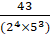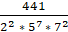Daily Practice Problems
Class 10 Maths
Real NumbersQuestion 1:

HCF and LCM of two numbers is 9 and 459 respectively. If one of the numbers is 27, find the other number.

Question 2:

Find LCM of numbers whose prime factorisation are expressible as 3 * 52 and 32 * 72.

Question 3:

Show that any positive odd integer is of the form 6q + 1, or 6q + 3, or 6q + 5, where q is some integer.

Question 4:

Find the largest number which divides 70 and 125 leaving remainder 5 and 8 respectively.

Question 5:

Find the HCF of the numbers 196 and 38220 using Euclid’s division algorithm.

Question 6:

Find the prime factorisation of the denominator of rational number expressed as 6.12 in simplest form.

Question 7:

Prove that √5 is irrational number.

Question 8:

Show that 3√7 is an irrational number.

Question 9:

Prove that 3 + 2√2 is an irrational number, given that √2 is an irrational number.

Question 10:

Three alarm clocks ring at intervals of 4, 12 and 20 minutes respectively. If they start ringing together, after how much time will they next ring together?

Question 11:

What is the smallest number which is exactly divisible by 40, 50 and 60?

Question 12:

Amita, Sneha, and Raghav start preparing cards for all persons of an old age home. In order to complete one card, they take 10, 16 and 20 minutes respectively. If all of them started together, after what time will they start preparing a new card together?

Question 13:

Express 429 as a product of its prime factors.

Question 14:

If HCF of 408 and 1032 is expressible in the form of 1032 * 2 + 408 * p, then find the value of p.

Question 15:

The HCF of 65 and 117 is expressible in the form 65p - 117. Find the value of p. Also find the LCM and HCF of 65 and 117 using prime factorisation method.

Question 16:

If p = HCF(8, 12) and q = LCM(8, 12), then find p2q.

Question 17:

Find the LCM and HCF of the integers 510 and 92 and verify that LCM * HCF = product of the two numbers.

Question 18:

The decimal expansion of the rational numberwill terminate after how many places of decimals?

Question 19:

Has the rational numberterminating?

Question 20:

A rational number in its decimal expansion is 1.7351. What can you say about the prime factors of q when this number is expressed in the form pq? Give reason.

**********